# Module 2: BASIC PROGRAMMING FUNDAMENTALS

## Learning Objectives

1. know the programming concept of a character/string,

2. use strings in MATLAB.

## What is a character?

A character, or “char” in MATLAB, is a data type (we will cover what “data types” are in Lesson 2.5) that is as simple as a one single unit: a text character. This can be a symbol, letter, punctuation, space, etc.

In MATLAB, you can turn text into characters by using single quotes (e.g., 's', 'g'). The text will then turn purple signifying that MATLAB now recognizes the text as characters, and has stored it that way.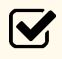Important Note: If it is a character, MATLAB does not recognize it as numbers, variables, functions, etc. For example, '2+2' as a string of characters does not equal 4. It is only text and will not be calculated.

### Example 1

Assign the characters “1”, “n”, and “x” to three different variables.

Solution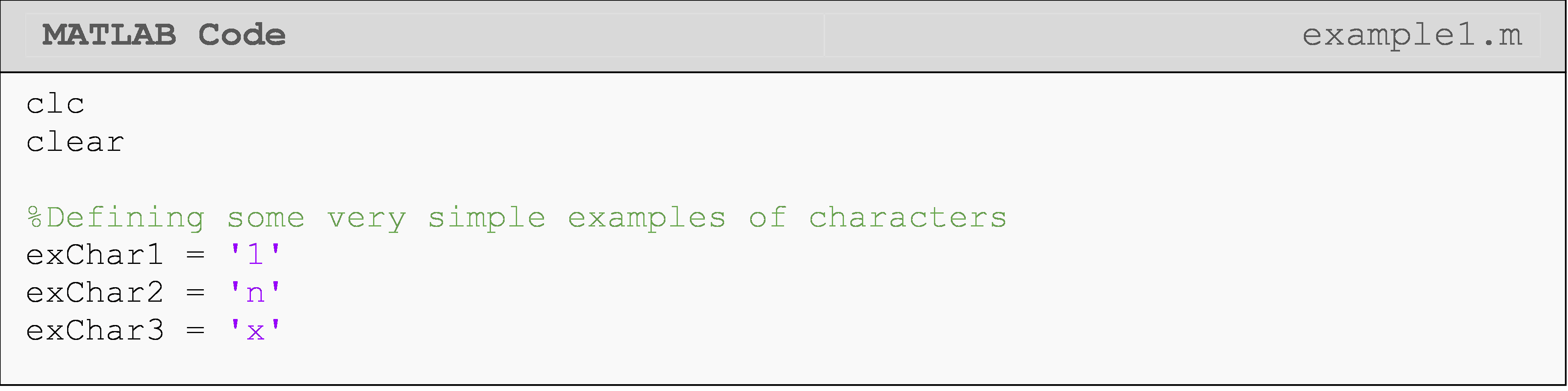Without recognizing the text as a character, MATLAB will think it is a variable and ask the computer for its value. You can verify this for yourself by removing the quotes on exChar2 or exChar3 (e.g., exChar2 = n).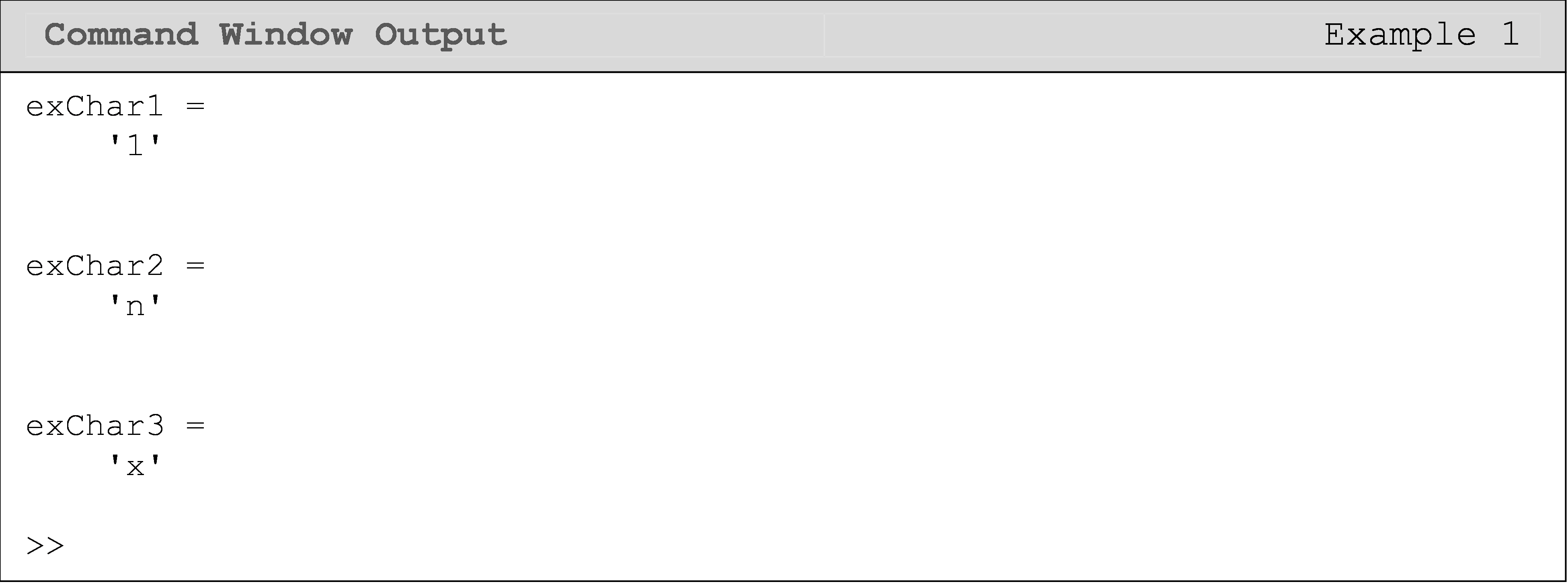## What is a string?

A string is an array of characters (alphanumeric) and can be a word, a sentence, or any other combination of characters. Strings are often used to display and describe outputs in the Command Window. In MATLAB, a string is established by the characters that are set within two single quotes (example: 'This is an example of string theory'). Strings can be assigned to a standard variable just like any other expression as shown in Example 2. They also have array “properties” like referencing specific portions of the string as seen in Example 2. We will cover this in more detail in Lesson 2.6: Vectors and Matrices. For now, just know that this is possible with strings.

### Example 2

Assign the text “Take 5!” to a variable.

Solution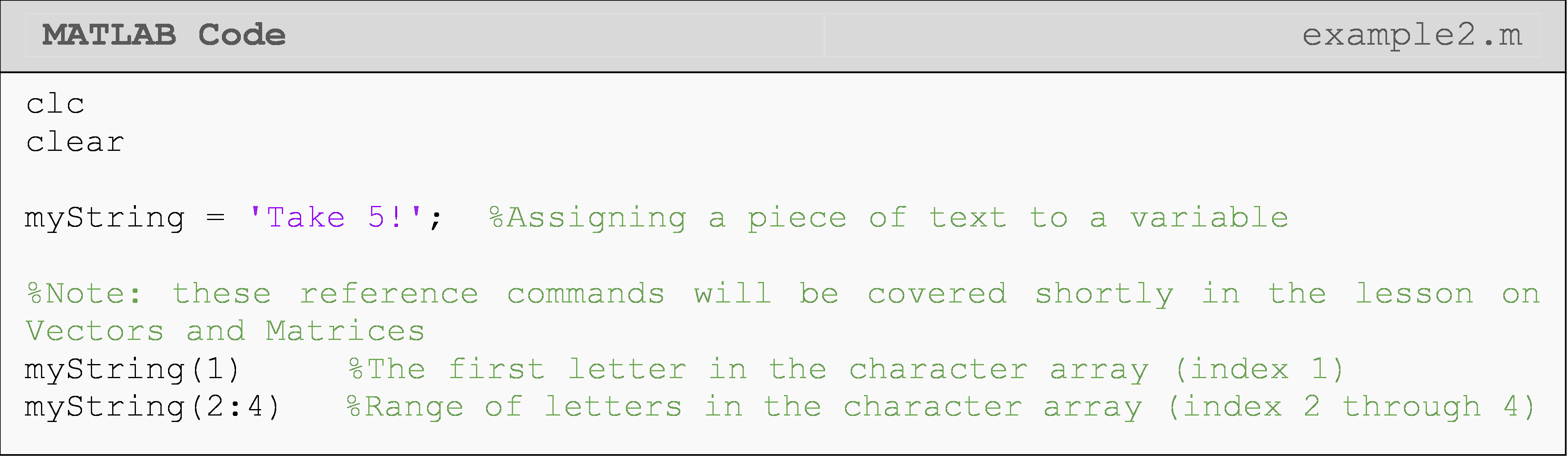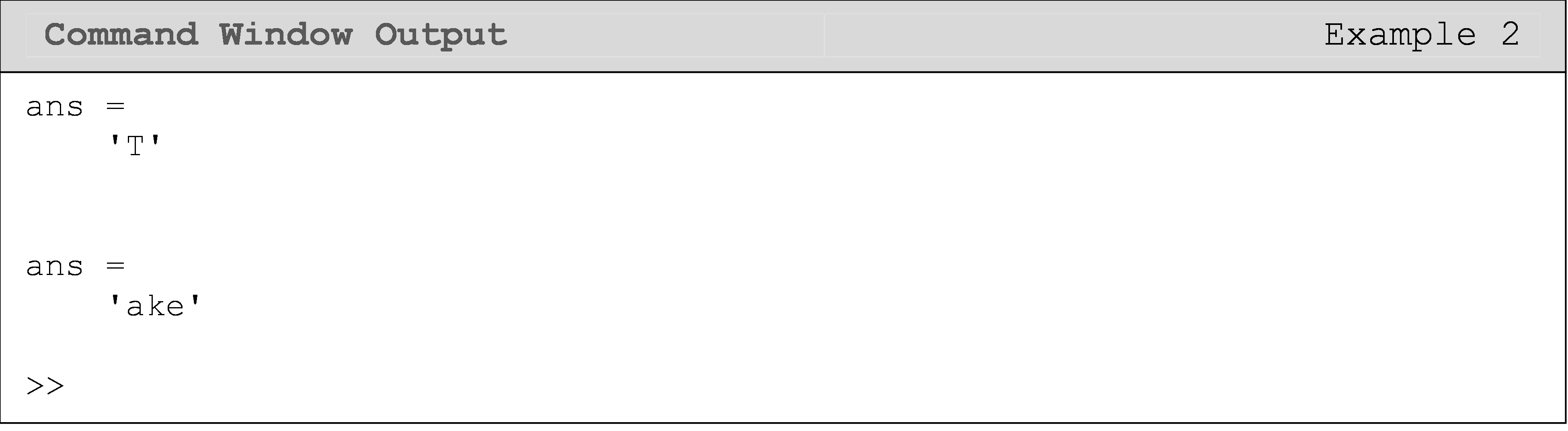## What makes characters/strings special?

Example 3 demonstrates some of the things we have talked about so far in this lesson. When using the characters, MATLAB will not recognize the text as variables, numbers, etc. This is important to remember going forward. The use of a variable in a string must be called in a special way, which we will learn in Lessons 2.3 and 2.5. Writing a variable name inside a string, as shown in the last line of Example 3, will not work!

### Example 3

Show examples of the properties of strings.

Solution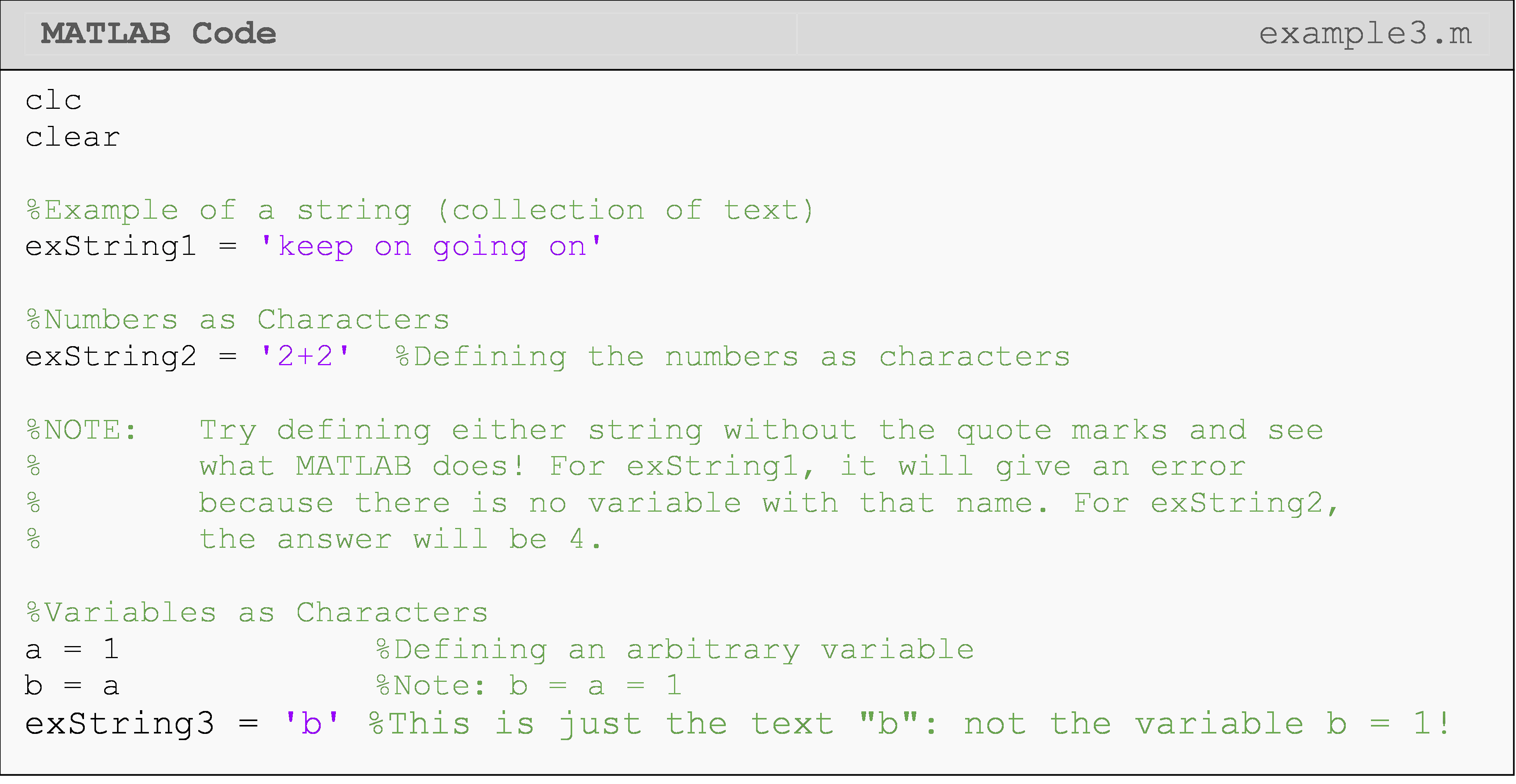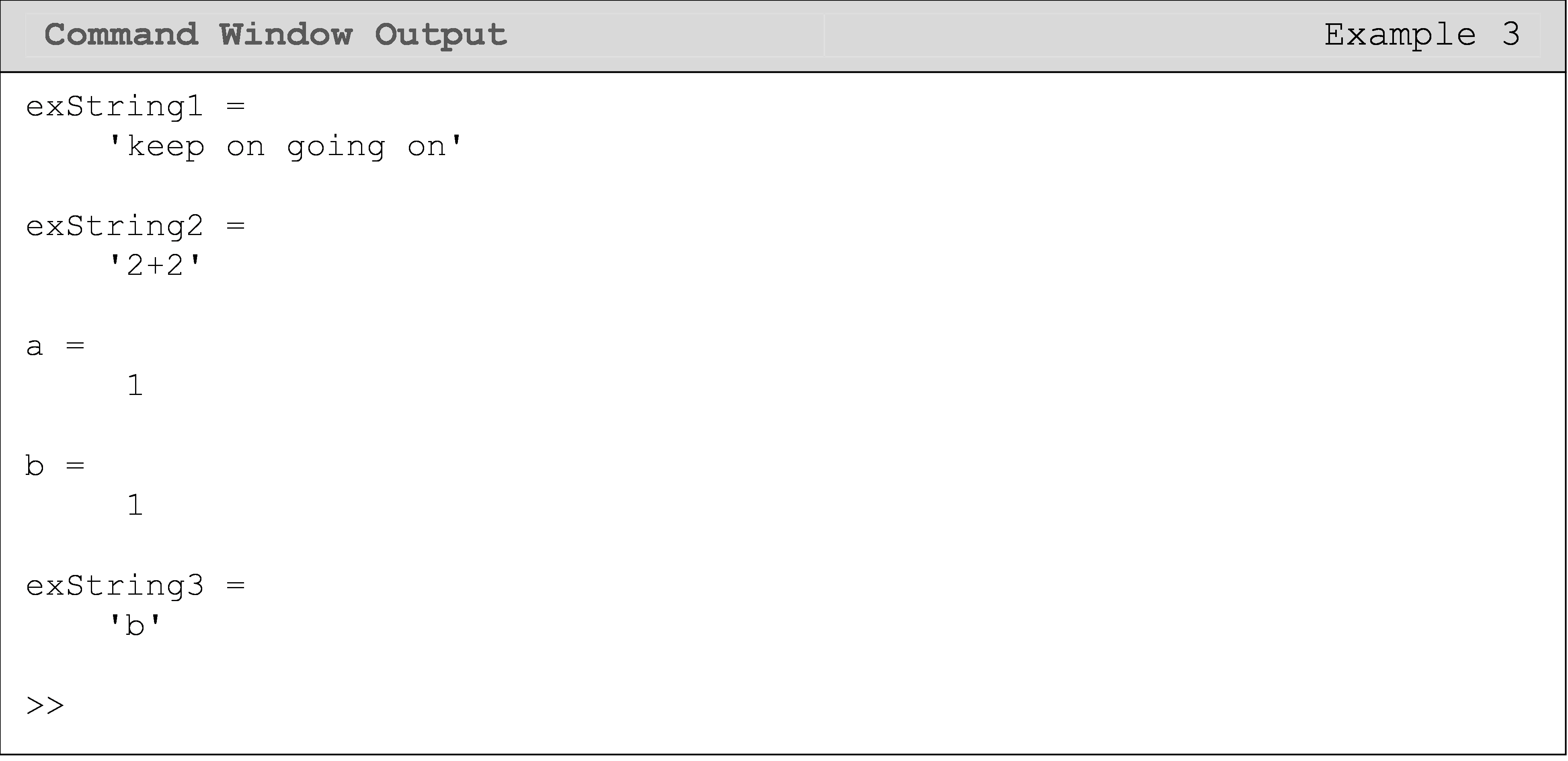## Lesson Summary of New Syntax and Programming Tools

 Task Syntax Example Usage Use text in the program 'some text' exStr = 'some text' Reference part of a string exStr(1:4) ans = 'some'

## Multiple Choice Quiz

(1). Strings are designated by using

(a) / /

(b) ' '

(c) \ \

(d) " "

(2). What is the Command Window output of the following code?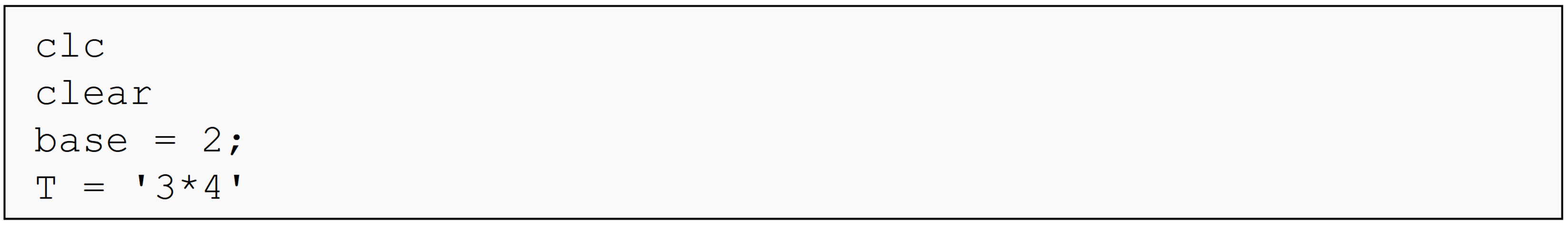(a)  base = 2;

(b)  T = '3*4'

(c)  T = 12

(d)  Nothing, there will be an error.

(3). Which of the following will perform the addition of the numbers 3 and 4 in MATLAB?

(a)  sum = (3 + 4)

(b)  sum = '3 + 4'

(c)  sum = '3' + '4'

(d)  All of the above

(4). What is the Command Window output of the following code?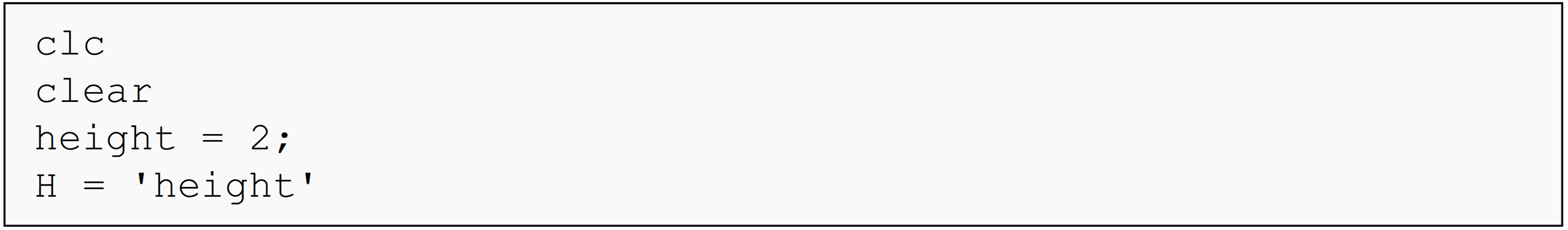(a)  H = 'height'

(b)  H = 2

(c)  Undefined function or variable 'height'.

(d)  None of the above

(5). To store the phrase “Land of the Free” in a variable, the correct code is

(a)  myVar! = 'Land of the Free!'

(b)  myVar = Land of the Free

(c)  myVar = 'Land of the Free'

(d)  'Land of the Free'

## Problem Set

1. Store the phrase “Sweet Home Alabama” in a variable and suppress the variable. Then call that variable and observe its output.

1. Write mathematical operations 7*(5+4) as a string and store in the variable myString. Next, complete the operations as mathematical numbers (answer should be 63) and store in the variable hwAnswer.

1. Use the characters % and ' in a string. Hint: since these are special characters in strings in MATLAB, use doubles of each character (e.g., %% and '').

1. Get the first name from the string 'John Smith', and store it in the variable firstName.

1. Get the last name from the string 'Jane Doe', and store it in the variable lastName.